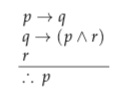# 2.5.1: 2.5.B Valid arguments and proofs

In general, arguments are more complicated that those we’ve considered so far. Here, for example, is an argument that has five premises:

(p r) → s

qp

tr
q

t

s

Is this argument valid? Of course, you could use a truth table to check whether the conjunction of the premises logically implies the conclusion. But with five propositional variables, the table would have 32 lines, and the size of the table grows quickly when more propositional variables are used. So, in general, truth tables are not practical when we have a large number of variables.

For a relatively small number of variables(say three or fewer) a truth table can be a rather efficient method to test validity of an argument. In one of the pencast of this course we show how you can use truth tables to test for validity as well as how you can use them to find counterexamples for invalid arguments: youtu.be/lSZS3qbA88o

Fortunately, there is another way to proceed, based on the fact that it is possible to chain several logical deductions together. That is, if P =⇒ Q and Q =⇒ R, it follows thatP =⇒ R. This means we can demonstrate the validity of an argument by deducing the conclusion from the premises in a sequence of steps. These steps can be presented in the form of a proof:

Definition 2.11.

A formal proof that an argument is valid consists of a sequence of pro- positions such that the last proposition in the sequence is the conclusion of the argument, and every proposition in the sequence is either a premise of the argument or follows by logical deduction from propositions that precede it in the list.

The existence of such a proof shows that the conclusion follows logically from the premises, and therefore that the argument is valid. Here is a formal proof that the argument given above is valid. The propositions in the proof are numbered, and each proposition has a justification.

Proof.Once a formal proof has been constructed, it is convincing. Unfortunately, it’s not necessarily easy to come up with the proof. Usually, the best method is a combination of working forward (“Here’s what I know, what can I de- duce from that?”) and working backwards (“Here’s what I need to prove, what other things would imply that?”). For this proof, I might have thought: I want to prove s. I know that p r implies s, so if I can prove p r, I’m okay. But to prove p r, it’ll be enough to prove p and r separately....

Of course, not every argument is valid, so the question also arises, how can we show that an argument is invalid? Let’s assume that the argument has been put into general form, with all the specific propositions replaced by propositional variables. The argu- ment is valid if in all cases where all the premises are true, the conclusion is also true. The argument is invalid if there is even one case where all the premises are true and the conclusion is false. We can prove that an argument is invalid by finding an assign- ment of truth values to the propositional variables which makes all the premises true but makes the conclusion false. We call such an assignment a counterexample . To disprove the validity of an argument you should always provide a counterexample. This holds in propositional logic, predicate logic, and any other type of argument you may be asked to disprove.

For example, consider an argument of the form:In the case where p is false, q is false, and r is true, the three premises of this argument are all true, but the conclusion is false. This counterexample shows that the argument is invalid.

To apply all this to arguments stated in English, we have to introduce propositional variables to represent all the propositions in the argument. For example, consider:

John will be at the party if Mary is there and Bill is not there. Mary will be at the party if it’s on Friday or Saturday. If Bill is at the party, Tom will be there. Tom won’t be at the party if it’s on Friday. The party is on Friday. Therefore, John will be at the party.

Let j stand for “John will be at the party”, m for “Mary will be there”, b for “Bill will be there”, t for “Tom will be there”, f for “The party is on Friday”, and s for “The party is on Saturday”. Then this argument has the formThis is a valid argument, as the following proof shows:

Proof.

1. f → ¬t premise

2. f premise

3. ¬t from 1 and 2 (modus ponens)

4. b t premise

5. ¬b from 4 and 3 (modus tollens)

6. fs from 2

7. (fs)→m premise

8. m from 6 and 7 (modus ponens)

9. m∧¬b from 8 and 5

10. (m∧¬b)→j premise

11. j from 10 and 9 (modus ponens)

You may have noticed that we start our proofs with the word ‘proof’ and end it with a little square. This is done to illustrate clearly where our proof starts and ends. Historically different symbols and expressions have been used to indicate that a proof is done. You may have heard of the abbreviation Q.E.D. for instance for ‘Quod Erat Demonstrandum’, which translates to: ‘what was to be shown’. Even in ancient Greece a Greek version of Q.E.D. was used by Greek mathematicians like Euclid. You are free to choose between Q.E.D. and the open square, so long as you remember that no proof is complete if it does not have either one of them.